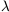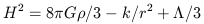### 10. IMPLICATIONS FOR COSMOLOGY

One of the classical tests of cosmology is the comparison of timescales. With a knowledge of H0 , the average density of matter,, and the value of the cosmological constant,, integration of the Friedmann equation(7)

yields a measure of the expansion age of the universe. This expansion age can be compared with other independent estimates of the age of the Galaxy and its oldest stars, t0, and thus offers a test of various possible cosmological models. For example, the dimensionless product, H0 t0, is 2/3 in the simplest case wherem = 1,= 0 (the Einstein-de Sitter model), and the product is 1 for the case of an empty universe where the matter and energy density are zero.

An accurate determination of the expansion age requires not only the value of H0 , but also accurate measurements ofm and. At the time when the Key Project was begun, the strong motivation from inflationary theory for a flat universe, coupled with a strong theoretical preference for= 0, favored the Einstein-de Sitter model (e.g., Kolb & Turner 1990). In addition, the ages of globular cluster stars were estimated at that time to be ~ 15 Gyr (VandenBerg, Bolte & Stetson 1996; Chaboyer et al. 1996). However, for a value of H0 = 72 km s-1 Mpc-1, as found in this paper, the Einstein-de Sitter model yields a very young expansion age of only 9 ± 1 Gyr, significantly younger than the globular cluster and other age estimates.

Over the past several years, much progress has been made toward measuring cosmological parameters, and the Einstein-de Sitter model is not currently favored. For example, estimates of cluster velocity dispersions, X-ray masses, baryon fractions, and weak lensing studies all have provided increasingly strong evidence for a low-matter-density (m) universe (e.g., Bahcall & Fan 1998). In addition, strong new evidence for a flat universe has emerged from measurements of the position of the first acoustic peak in recent cosmic microwave background anisotropy experiments (de Bernardis et al. 2000; Lange et al. 2000). Together with evidence for a low matter density, and with recent data from high-redshift supernovae (Riess et al. 1998; Perlmutter et al. 1999), evidence for a non-zero cosmological constant has been increasing. Moreover, the age estimates for globular clusters have been revised downward to 12-13 Gyr, based on a new calibration from the Hipparcos satellite (Chaboyer 1998; Carretta et al. 2000). A non-zero value of the cosmological constant helps to avoid a discrepancy between the expansion age and other age estimates. For H0 = 72 km s-1 Mpc-1,m = 0.3,= 0.7, the expansion age is 13 ± 1 Gyr, consistent to within the uncertainties, with recent globular cluster ages. In Table 15, we show expansion ages for different values of H0 and a range of flat models.In Figure 9 H0t0 is plotted as a function of. Two curves are shown: the solid curve is for the case where= 0, and the dashed curve allows for non-zerounder the assumption of a flat universe. The ± 1- and 2-limits are plotted for H0 = 72 km s-1 Mpc-1, t0 = 12.5 Gyr, assuming independent uncertainties of ±10% in each quantity, and adding the uncertainties in quadrature. These data are consistent with either a low-density (m ~ 0.1) open universe, or a flat universe withm ~ 0.35,= 0.65; however, with these data alone, it is not possible to discriminate between an open or flat universe. As described above, recent studies favortotal = 1, a low-matter-density universe (m ~ 0.3), and a non-zero value of the cosmological constant. Note, however, that the open circle atm = 1,= 0, represents the Einstein-de Sitter case, and is inconsistent with the current values of H0 and t0 only at a ~ 2-level.Figure 9. H0t0 versusfor H0 = 72 km s-1 Mpc-1, t0 = 12.5 Gyr, and uncertainties of ± 10% adopted for both quantities. The dark line indicates the case of a flat Universe with+m = 1. The abscissa in this case corresponds to. The lighter curve represents a Universe with= 0. In this case, the abcissa should be read asm. The dashed and dot-dashed lines indicate 1-and 2-limits, respectively for values of H0 = 72 and t0 = 12.5 Gyr in the case where both quantities are assumed to be known to ±10% (1-). The large open circle denotes values of H0t0 = 2/3 andm = 1 ( i.e., those predicted by the Einstein-de Sitter model). On the basis of a timescale comparison alone, it is not possible to discriminate between models withm ~ 0.1,= 0 orm ~ 0.35,~ 0.65.